# Practice Exercises for Mathematical Logic

1.
 p q ~p p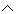q p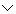q p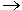q T T T T T T F F T F F T T F T F F T F F

 Enter Clear Feedback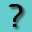2.
 p q ~q pq (pq)~q T F T F T F F T F T F T F F T F

 Enter Clear Feedback3.
 x y xy yx (xy)(yx) x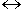y T T T T T T F F F F F T T F F F F T T T

 Enter Clear Feedback4.
 Which of the following statements from problem 3 is conditional? xyxyNone of the above. RESULTS BOX:5.
 Which of the following statements from problem 3 is biconditional? xyyxx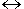yNone of the above. RESULTS BOX:

6.
 a b ab (ab)a [(ab)a]b T T T T T F F T F T T T F F T F

 Enter Clear Feedback7.
 Choose the word that best completes this sentence: The statement in the last column of the truth table in problem 6 is a ____________. BiconditionalTautologyDisjunctionNone of the above. RESULTS BOX:

8.
 p q ~q p~q pq ~(pq) (p~q)[~(pq)] T T F T F T T F T T T T F T T T F T F F T T F T

 Enter Clear Feedback9.
 Which two statements from problem 8 are logically equivalent? p~q and pqpq and ~(pq)p~q and ~(pq)None of the above. RESULTS BOX:10.
 Choose the word that best completes this sentence: The ____________ of two equivalent statements always yields a tautology. BiconditionalConjunctionNegationAll of the above. RESULTS BOX:

 Lessons on Symbolic Logic Negation Conjunction Disjunction Conditional Compound Biconditional Tautologies Equivalence Practice Exercises Challenge Exercises Solutions